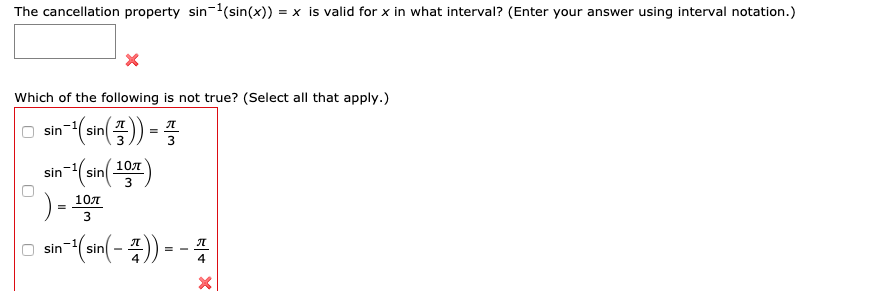# The cancellation property sin(sin(x))= x is valid for x in what interval? (Enter your answer using interval notation.)Which of the following is not true? (Select all that apply.)(an))sinsin310Tsinsin3103n(an(-4)лsinsin-

Question
1181 views

The cancellation property

sin−1(sin(x)) = x

is valid for x in what interval? (Enter your answer using interval notation.)help_outlineImage TranscriptioncloseThe cancellation property sin(sin(x)) = x is valid for x in what interval? (Enter your answer using interval notation.) Which of the following is not true? (Select all that apply.) (an)) sin sin 3 10T sin sin 3 10 3 n(an(-4) л sin sin - fullscreen
check_circle

star
star
star
star
star
1 Rating
Step 1

Given that

Step 2

We need to determine the interval of x for which the above cancellation property is valid.

We know that the range of sinx is

Step 3

So x lies in

&n...

### Want to see the full answer?

See Solution

#### Want to see this answer and more?

Solutions are written by subject experts who are available 24/7. Questions are typically answered within 1 hour.*

See Solution
*Response times may vary by subject and question.
Tagged in
MathTrigonometry

### Trigonometric Ratios